# Covering homotopy

(diff) ← Older revision | Latest revision (diff) | Newer revision → (diff)

for a homotopy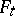of a mapping, given a mapping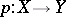A homotopy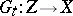such that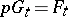. In this situation, if the covering mapping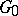for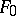is prescribed in advance, one says thatextends. The covering homotopy axiom, in its strong version, requires that, for a given mapping, for any homotopy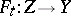from a paracompactum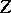and for any(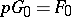), an extension ofto a covering homotopy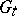exists. In that caseis said to be a Hurewicz fibration. The most important example is provided by the locally trivial fibre bundles (cf. Locally trivial fibre bundle). If the covering homotopy property is only required to hold in the case thatis a finite polyhedron,is called a Serre fibration.

Letandbe arcwise connected and let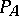be the path space of(i.e. the space of continuous mappings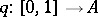). Consider a continuous mapping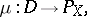whereand assume that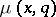begins at a pointand covers. Then the formula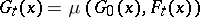yields an extension ofto a covering homotopy. In particular, a mappingsatisfying these conditions can be defined naturally for a covering, and also for a smooth vector bundle with a fixed connection. The validity of the covering homotopy axiom in Serre's formulation makes it possible to construct the exact homotopy sequence of a fibration (see Homotopy group).